# Irregular singular point

(diff) ← Older revision | Latest revision (diff) | Newer revision → (diff)
A concept that arose in the analytic theory of linear ordinary differential equations. Letbe an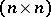-matrix that is holomorphic in a punctured neighbourhood ofand that has a singularity at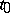.
The pointis then called a singular point of the system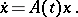(*)
There are two non-equivalent definitions of an irregular singular point. According to the first one,is called an irregular singular point of (*) if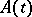has a pole of order greater than one at(cf. Analytic theory of differential equations, as well as ). According to the second definition,is called an irregular singular point of (*) if there is no number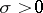such that every solution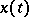grows not faster than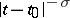asalong rays (cf. ). The case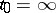can be reduced to the caseby the transformation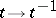. An irregular singular point is sometimes called a strongly-singular point (cf., e.g., Bessel equation). In a neighbourhood of an irregular singular point the solutions admit asymptotic expansions; these were studied by H. Poincaré for the first time .